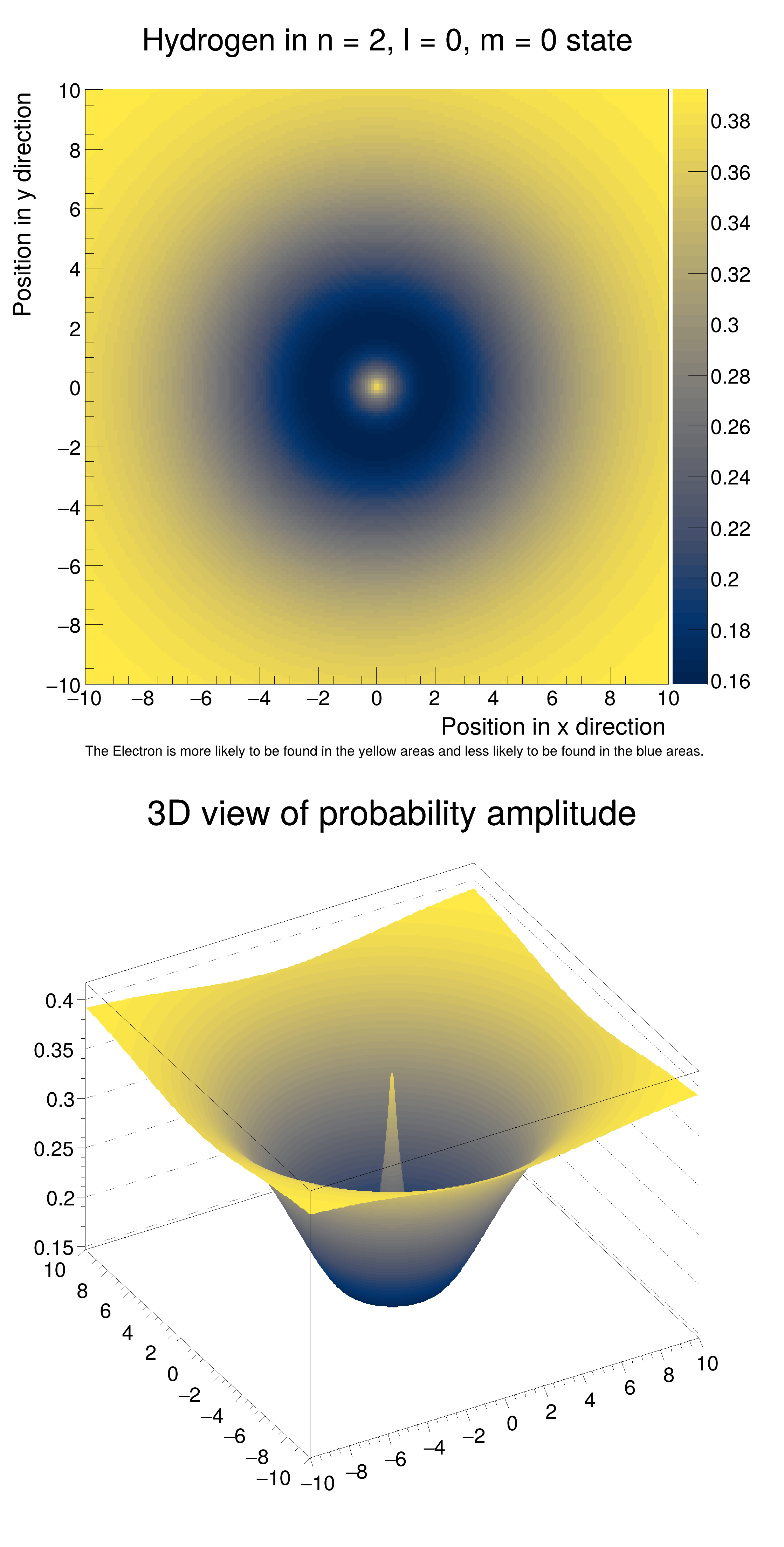ROOT   Reference Guideschroedinger_hydrogen.C File Reference

## Detailed DescriptionPlot the Amplitude of a Hydrogen Atom.

Visualize the Amplitude of a Hydrogen Atom in the n = 2, l = 0, m = 0 state. Demonstrates how TH2F can be used in Quantum Mechanics.

The formula for Hydrogen in this energy state is $$\psi_{200} = \frac{1}{4\sqrt{2\pi}a_0 ^{\frac{3}{2}}}(2-\frac{\sqrt{x^2+y^2}}{a_0})e^{-\frac{\sqrt{x^2+y^2}}{2a_0}}$$#include <cmath>
double WaveFunction(double x, double y) {
double r = sqrt(x *x + y*y);
double w = (1/pow((4*sqrt(2*TMath::Pi())* 1), 1.5)) * (2 - (r / 1)*pow(TMath::E(), (-1 * r)/2)); // Wavefunction formula for psi 2,0,0
return w*w; // Amplitude
}
void schroedinger_hydrogen() {
TH2F *h2D = new TH2F("Hydrogen Atom",
"Hydrogen in n = 2, l = 0, m = 0 state; Position in x direction; Position in y direction",
200, -10, 10, 200, -10, 10);
for (float i = -10; i < 10; i += 0.01) {
for (float j = -10; j < 10; j += 0.01) {
h2D->Fill(i, j, WaveFunction(i, j));
}
}
TCanvas *c1 = new TCanvas("c1", "Schroedinger's Hydrogen Atom", 750, 1500);
c1->Divide(1, 2);
auto c1_1 = c1->cd(1);
c1_1->SetRightMargin(0.14);
h2D->GetXaxis()->SetLabelSize(0.03);
h2D->GetYaxis()->SetLabelSize(0.03);
h2D->GetZaxis()->SetLabelSize(0.03);
h2D->SetContour(50);
h2D->Draw("colz");
TLatex *l = new TLatex(-10, -12.43, "The Electron is more likely to be found in the yellow areas and less likely to be found in the blue areas.");
l->SetTextFont(42);
l->SetTextSize(0.02);
l->Draw();
auto c1_2 = c1->cd(2);
c1_2->SetTheta(42.);
TH2D *h2Dc = (TH2D*)h2D->Clone();
h2Dc->SetTitle("3D view of probability amplitude;;");
h2Dc->Draw("surf2");
}
ROOT::R::TRInterface & r
Definition: Object.C:4
@ kCividis
Definition: TColor.h:127
double pow(double, double)
double sqrt(double)
R__EXTERN TStyle * gStyle
Definition: TStyle.h:412
virtual void SetLabelSize(Float_t size=0.04)
Set size of axis labels.
Definition: TAttAxis.cxx:205
The Canvas class.
Definition: TCanvas.h:23
virtual void SetTitle(const char *title)
Definition: TH1.cxx:6680
TAxis * GetZaxis()
Definition: TH1.h:322
TAxis * GetXaxis()
Definition: TH1.h:320
TObject * Clone(const char *newname=0) const
Make a complete copy of the underlying object.
Definition: TH1.cxx:2741
TAxis * GetYaxis()
Definition: TH1.h:321
virtual void SetContour(Int_t nlevels, const Double_t *levels=0)
Set the number and values of contour levels.
Definition: TH1.cxx:8326
virtual void Draw(Option_t *option="")
Draw this histogram with options.
Definition: TH1.cxx:3074
2-D histogram with a double per channel (see TH1 documentation)}
Definition: TH2.h:292
2-D histogram with a float per channel (see TH1 documentation)}
Definition: TH2.h:251
Int_t Fill(Double_t)
Invalid Fill method.
Definition: TH2.cxx:358
To draw Mathematical Formula.
Definition: TLatex.h:18
void SetOptStat(Int_t stat=1)
The type of information printed in the histogram statistics box can be selected via the parameter mod...
Definition: TStyle.cxx:1589
void SetPalette(Int_t ncolors=kBird, Int_t *colors=0, Float_t alpha=1.)
See TColor::SetPalette.
Definition: TStyle.cxx:1782
return c1
Definition: legend1.C:41
Double_t y[n]
Definition: legend1.C:17
Double_t x[n]
Definition: legend1.C:17
constexpr Double_t E()
Base of natural log:
Definition: TMath.h:96
constexpr Double_t Pi()
Definition: TMath.h:37
auto * l
Definition: textangle.C:4

Definition in file schroedinger_hydrogen.C.